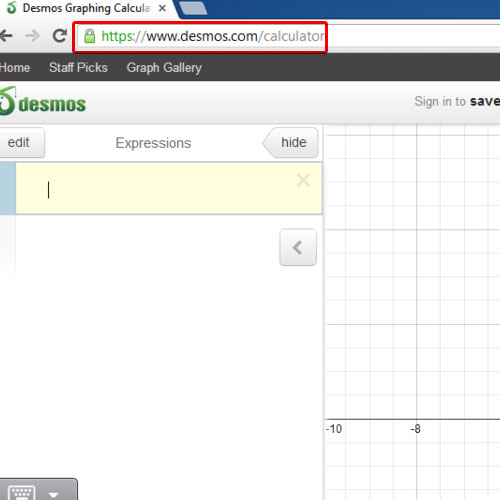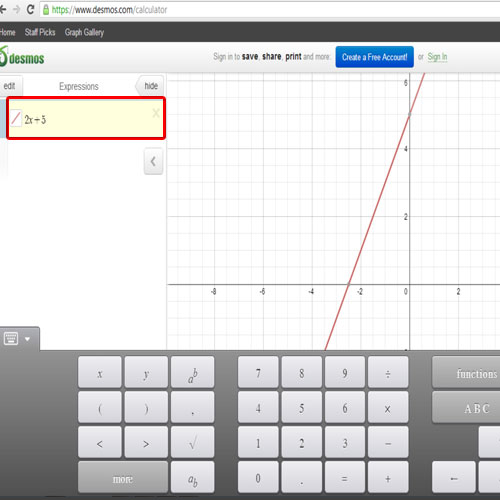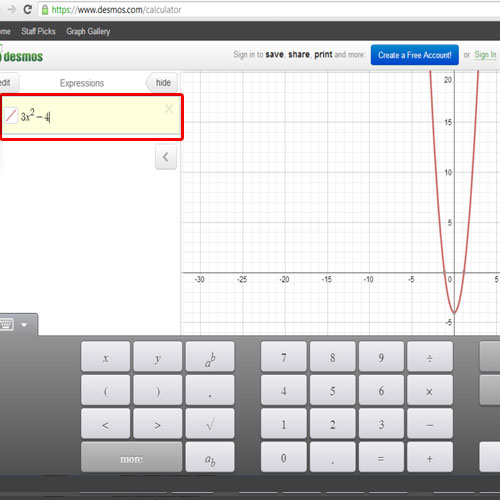## How to Use Free Graphing Calculator Online

In this tutorial, we will teach you how to use a free graphing calculator online. You can enter the expression of your choice and make a graphical representation of it. We will draw the graph of a straight line. Also, we will enter a quadratic expression and make the curve of the expression.

Step 1 – Open desmos.com

Follow this step by step guide to learn how to use a free graphing calculator online.
First of all, open a web browser of your choice and go to desmos.com/calculator.Step 2 – Draw a straight line

Once the web page loads, you will find the graph calculator in the middle of the page. You can enter the expression on the left hand side and the graph will be calculated for that very expression. On the bottom of the page, you have the expression variables, typical calculator and functional keys. We will draw a straight line first. For that, we will feed an expression along the lines of y = mx + c; the slope and the y-intercept clearly defined.Step 3 – Draw a curve

You can always clear the expression and draw the graph for another one. Next, we will try to experiment with a curve. We will use the expression 3x² – 4 and the graph for the respective expression will appear.
In this manner, you can use the graph calculator for drawing a straight line, a curve or any other expression.